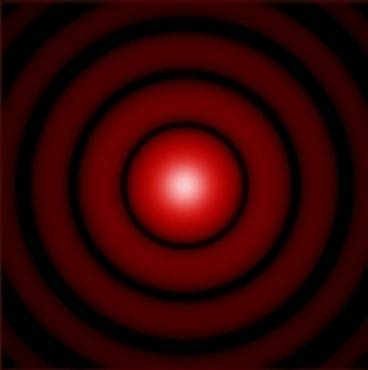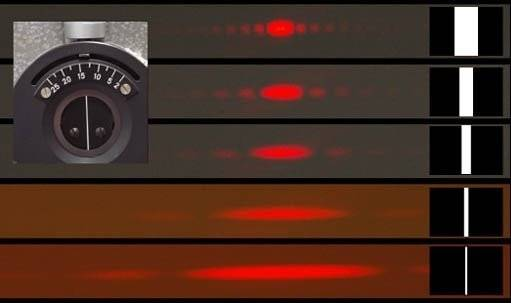# Fraunhofer diffraction

Hi,
Why is that when a diffraction pattern is created through small circular opening you achieve a diffraction pattern like this;But when we see images of a slot we see this;From; http://hyperphysics.phy-astr.gsu.edu/hbase/phyopt/fraungeo.html#c1

Notice how the diffraction is linear - not vertical or circular. If the same method is used in calculating the slot diffraction as you use in the circle then then slot diffraction should also be radial (but stretched to account for the slot height),

At first I thought this was because of the laser polarisation but since a laser also creates the circular image then this must not be the case??

Drakkith
Staff Emeritus
Yes, the smaller the width of the slot, the larger the diffraction pattern. A circular pattern behaves the same way. The smaller the circle, the larger the pattern. The slot gives a increased x-axis spread when the x-axis of the slot (width) is smaller because there is less destructive interference along that axis. The Y-axis remains the same size because the height of the slot remains unchanged. A circular aperture changes size in both axes, so the diffraction pattern does so too.

Yes - I agree but....

In the slot images of my first post the slit is either short or the width of the laser beam is visible - we can tell this from the fuzzy fringes in the y-axis. Note there is no interference indicated above and below the slit - but there should be given the width of the fringes in the x-axis and y-axis are comparable - so there should be intense light above and below that is missing....?

Also, as you say, as the width of the slot increases the interference pattern should become closer together. Consider now rotating the x - axis distribution function incrementally around to the y-axis position. If the aperture is a circle, we can see how this x-axis rotation of the probability distribution would create a circular airy's style image. But if we make the opening a slit - as we rotate the prob distribution the apparent slit width increases, bringing the fringes closer together - so shouldn't we see some curving on the top and bottom of the interference pattern?

#### Attachments

sophiecentaur
Gold Member
If you want to get a feel for the way that Diffraction works, there is no substitute for the mathematical approach. The two slits experiment is almost explained using very basic geometry, to account for the cancellation and enhancement of the waves in different places. Once you get to calculating and explaining diffraction of an aperture of finite size you cannot avoid Integration. The language of non-maths just cannot cope with what the language of maths can say, here. What you can do, however, is learn to recognise some of the patterns and basic rules - as written above. The list of threads that also discuss this subject are at the bottom of this page.

•M Quack
Claude Bile
"If the same method is used in calculating the slot diffraction as you use in the circle then then slot diffraction should also be radial (but stretched to account for the slot height)"

Not so. It makes sense for a circular aperture to have a diffraction pattern with circular symmetry and a linear slot to have one with cartesian symmetry. Diffraction patterns in the far field can be calculated by taking the 2D Fourier transform of the aperture function (transmission as a function of position).

Claude.

Drakkith
Staff Emeritus
In the slot images of my first post the slit is either short or the width of the laser beam is visible - we can tell this from the fuzzy fringes in the y-axis. Note there is no interference indicated above and below the slit - but there should be given the width of the fringes in the x-axis and y-axis are comparable - so there should be intense light above and below that is missing....?

Looks to me like the height of the slit is larger than the diameter of the laser beam. (Isn't the picture that's inset on the top left a picture of the slit?)

But if we make the opening a slit - as we rotate the prob distribution the apparent slit width increases, bringing the fringes closer together - so shouldn't we see some curving on the top and bottom of the interference pattern?

I don't know what you're trying to say here. What is "apparent slit width" and how is it increasing?

sophiecentaur